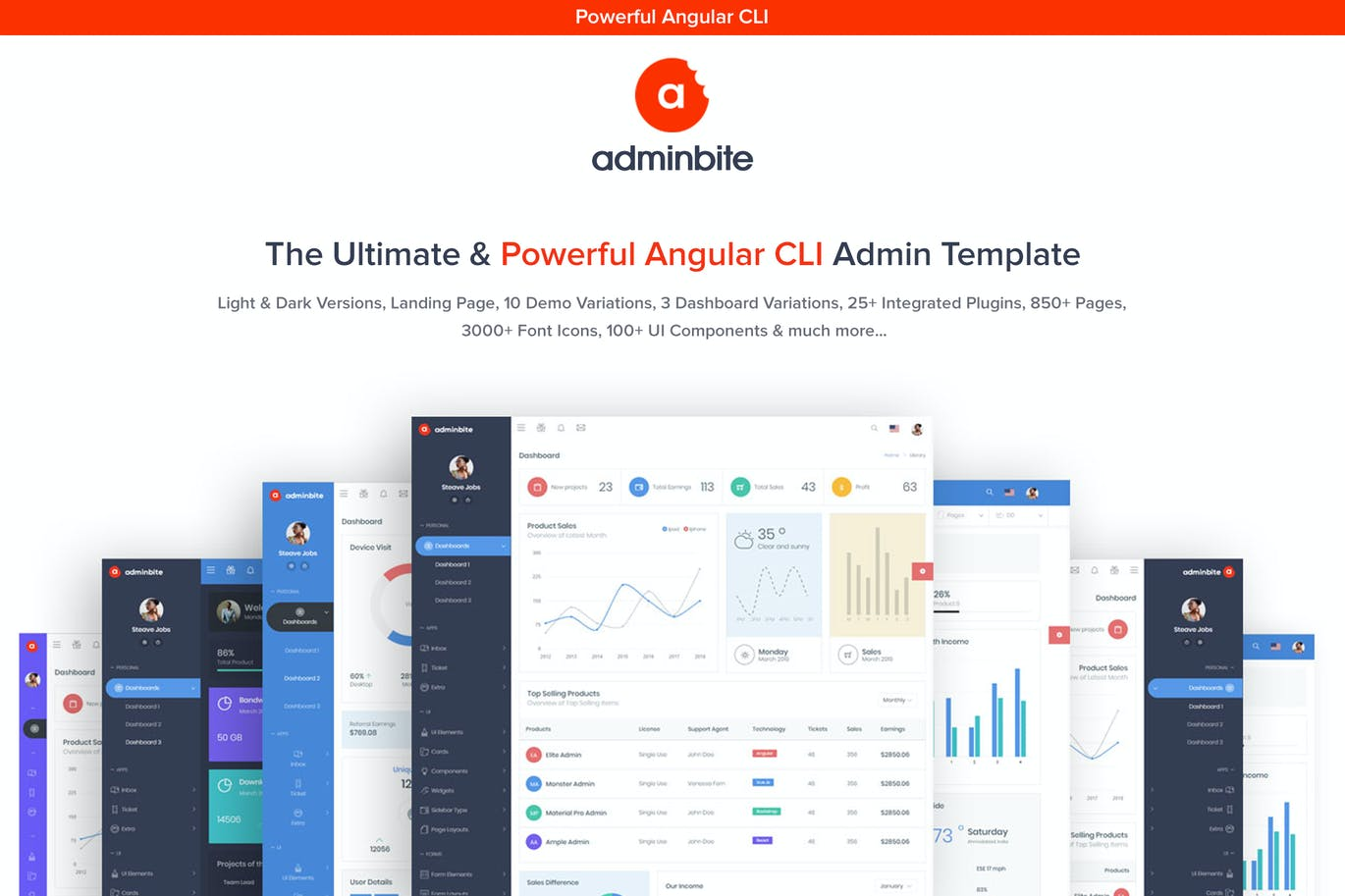• 3+不同的仪表盘
• 10种不同的演示
• Angular 6 / CLI
• 6种预定义的颜色皮肤
• 包括清洁和创意的着陆页（免费\$ 18）
• 浅色和深色配色方案
• 850多页
• 100多个UI组件
• 很多小部件
• 3000+字体图标
• 完全响应的页面
• SaSS基本CSS
• 易于定制
• 许多图表选项
• 多文件上传
• 许多表示例
• 验证表格
• 数据表

• 信箱
• 收件箱详细信息
• 撰写邮件
• 日历（新）
• 任务板（新）

UI元素

• 纽扣
• 烤面包机
• 手风琴
• 标签
• 编辑
• 时间线
• 落下
• 多文件上传
• 模态
• 进度条
• 通知事项
• 轮播
• 计价方式
• 工具提示
• 分页
• 等级
• 时间选择器（新）
• 拖曳 下降
• 发票（新）

• 基本形式
• 表格验证
• 表格字头
• 日期选择器

• 实用程序类（新）
• 登录页面
• 动画（新）
• 轮廓
• 发票
• 价钱
• 寄存器
• 恢复密码
• 错误404

• 图表js
• 查蒂斯

• 基本表
• 智能表（新）
• 数据表

• 字体很棒
• 主题图标
• 简单的线条图标